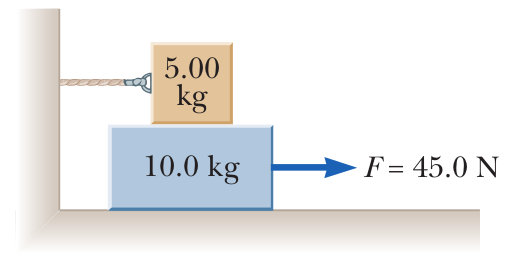# Problem: A 5.00-kg block is placed on top of a 10.0-kg block as shown in the figure. A horizontal force of 45.0 N is applied to the 10-kg block, and the 5.00-kg block is tied to the wall. The coefficient of kinetic friction between all moving surfaces is 0.200.(a) Draw a free-body diagram for each block and identify the action–reaction forces between the blocks.(b) Determine the tension in the string.(c) Determine the magnitude of the acceleration of the 10.0-kg block.

###### FREE Expert Solution

We're asked to determine the tension in the string attached to the top block and magnitude of the acceleration of the bottom block, as well as identifying action-reaction force pairs.

This is a system-of-objects type problem: Stacked Blocks With Friction. We'll follow these steps to solve:

1. Draw a free-body diagram for each body we're interested in
2. Write our Newton's Second Law Equations F=ma
3. Solve for the target variable

Obviously, we'll be using the equation for Newton's Second Law:

$\overline{)\begin{array}{rcl}\mathbf{\sum }\stackrel{\mathbf{⇀}}{\mathbit{F}}& {\mathbf{=}}& \mathbit{m}\stackrel{\mathbf{⇀}}{\mathbit{a}}\end{array}}$

We'll also need to relate the normal force and kinetic friction force:

$\overline{){{\mathbit{f}}}_{{\mathbit{k}}}{\mathbf{=}}{{\mathbit{\mu }}}_{{\mathbit{k}}}{\mathbit{N}}}$

93% (203 ratings)###### Problem Details

A 5.00-kg block is placed on top of a 10.0-kg block as shown in the figure. A horizontal force of 45.0 N is applied to the 10-kg block, and the 5.00-kg block is tied to the wall. The coefficient of kinetic friction between all moving surfaces is 0.200.

(a) Draw a free-body diagram for each block and identify the action–reaction forces between the blocks.

(b) Determine the tension in the string.

(c) Determine the magnitude of the acceleration of the 10.0-kg block.